# KS2 (key stage 2) Online Venn diagrams Quiz

#### Quiz Description

KS2 (key stage 2) Online Venn diagrams Quiz

Revising Venn diagrams with quizzes.

(KS2 Venn diagrams quizzes for pupils preparing for their end of term exams or children that wish to better prepare of the end of year KS2 SATs exams)

Welcome to gcequiz.com, your best partner for revising for your past ks2 exams. Today we will look into venn diagrams, what is it?,and how our quizzes help in  studying venn diagrams.

To fully understand what the Venn diagram is all about, you need to understand what SETS are. A Set is simply a collection or grouping of well defined items, we have as an example; a set of girls, a set of tea cups, a set of counting numbers less than 5 etc. Sets are subdivided into finite and infinite sets.

A Venn diagram is therefore a diagrammatic representation of two or more sets (That is, overlapping circles) outlining the differences between them.

What are the prerequisites or requirements to draw a set?

To draw a Venn Diagram, we need to know

1. The union of the sets involved. That is, all the elements present in these sets.
2. The intersection of these sets should also be known. This helps us to figure out what these sets have in common.

Generally, Venn diagrams help students in  ks2  to sort data. The data can be of any type: numbers, shapes, colors and so on. Sorting helps ks2 to distinguish between different object types. For example, children may be given a set of colors and be asked to separate them in two groups. Equally they may be given a set of numbers and be asked to group them in even and odd numbers.

To conclude, venn diagrams have an important part in the KS2  exams and also in children's personal growth. With that said, our platform has put in place a set of quizzes that can make students better understand venn diagrams, if you think you are ready for them then, select them below and start.

Play, Learn and have Fun!

What is A n B?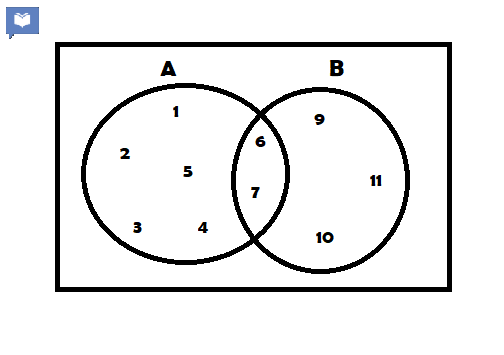Correct
• {6, 7}
• {9, 10, 11}
• {1, 2, 3, 4, 5}
• None of the above

List all the elements in ACorrect
• {6,7}
• {1, 2, 3, 4, 5}
• {1,2,3,4,5,6,7}
• {9,10,11}

List all the elements in BCorrect
• {6,7}
• {1,2,3,4,5,6,7}
• {9,10,11}
• {6,7,9,10,11}

What is A u B ?Correct
• {1,2,3,4,5,6,7}
• {6,7,8,9,10,11}
• {1,2,3,4,5,6,7,9,10,11}
• {1,2,3,4,5,6,7,8,9,10,11}

Which elements are in A only ?Correct
• {6,7}
• {1,2,3,4,5}
• {1,2,3,4,5,6,7}
• {1,2,3,4,5,6,7,8}

Which elements are in B only ?Correct
• {9,10,11}
• {6,7,9,10,11}
• {1,2,3,4,5}
• {1,2,3,4,5,6,7,8,9,10,11}

List all elements in X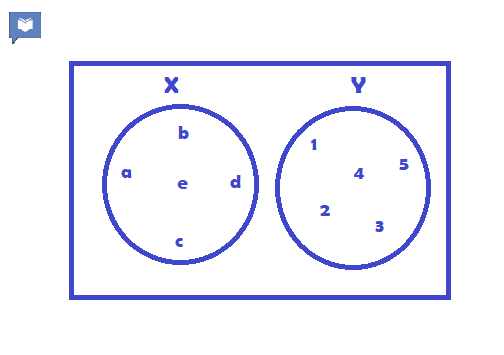Correct
• {a,b,c,d,e}
• {1,2,3,4,5}
• {a,2,3,4,d,e}
• {1,2,3,4,5,a,b,c,d,e}

List all elements in YCorrect
• {a,b,c,d,e}
• {1,2,3,4,5}
• {1,2,3,d,e}
• {1,2,3,4,5,6,7}

What is A n B ?Correct
• {a,b}
• {1,2}
• {a,b,1,2}
• { }

A n B is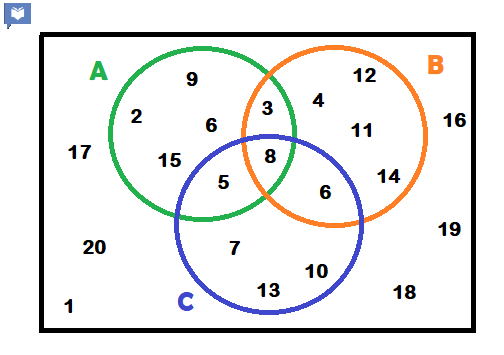Correct
• {3}
• {8}
• {5}
• {6}

B n C isCorrect
• {3}
• {6}
• {5}
• {8}

A n C isCorrect
• {3}
• {5}
• {6}
• {8}

What is A n B n C ?Correct
• {3}
• {5}
• {6}
• {8}

What is P '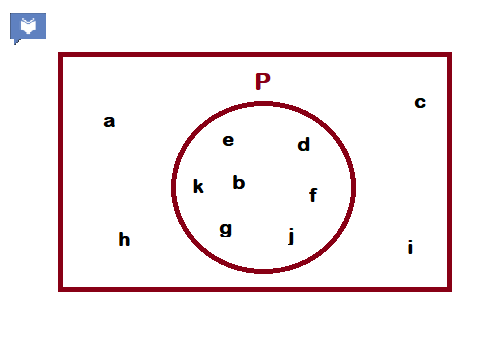Correct
• {a,b,c,d,e,f)}
• {b,c,d,e,f,g,h}
• { }
• {a,c,h,i}

What is B ' ?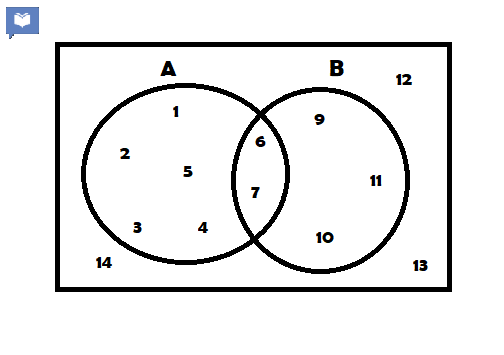Correct
• {1,2,3,4,5}
• {1,2,3,4,5,6,7,12,13,14}
• {1,2,3,4,5,12,13,14}
• { }